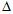# Electrical Engineering - Three-Phase Systems in Power Applications

### Exercise :: Three-Phase Systems in Power Applications - True or False

1.

In a-connected generator, there is a 120° difference between each line current and the nearest phase current.

 A. True B. False

Explanation:

No answer description available for this question. Let us discuss.

2.

Power is measured in a three-phase load by using an ammeter.

 A. True B. False

Explanation:

No answer description available for this question. Let us discuss.

3.

Polyphase is characterized by two or more sinusoidal voltages, each having a different phase angle.

 A. True B. False

Explanation:

No answer description available for this question. Let us discuss.

4.

In a Y-connected generator, there is a 120° difference between each line voltage and the nearest phase voltage.

 A. True B. False

Explanation:

No answer description available for this question. Let us discuss.

5.

A simple three-phase generator consists of three conductive loops separated by 120°.

 A. True B. False